# Finding Optimal Routes for Main Haul Roads Development in Mountainous Open Pit using A* algorithm

Kun Ui Hong1, Un Chol Han2*, Mun Hyok Kim1, Chung Il Kim1 and Bun Hui Kim3

1Faculty of Mining Engineering, Kim Chaek University of Technology, Pyongyang 999093, Democratic People’s Republic of Korea

2School of Science and Engineering, Kim Chaek University of Technology, Pyongyang 999093, Democratic People’s Republic of Korea

3Faculty of Application mathematics, Kim Chaek University of Technology, Pyongyang 999093, Democratic People’s Republic of KoreaUniversity of Lisbon, Portugal

Received Date: June 02, 2022;  Published Date: June 21, 2022

#### Abstract

The main haul roads can significantly affect the cost associated with hauling ore and waste to the concentrating mill or waste dump, so finding optimal routes for main haul roads development is very important for opening-up the mountainous open pit. In this study, we propose a new method to search the optimal route for main haul roads development using A* algorithm based on a 3D terrain model of the mountainous surface mine. Here, a 3D terrain model was made by using Geovia Surpac software and input data for finding optimal routes were prepared by processing string file of Surpac and extracting the 3D coordinates in the mine area. The haul road layout in the mountainous surface mine was optimized by using A* algorithm based on least-cost path analysis. Finally, a 3D model reflecting the result of the main haul road layout was created by combining the haul road layout result with a 3D topographic model of the mountainous surface mine. The proposed approach was applied to the main haul road design for a limestone mine of Kangdong cement factory, DPR Korea. As a result, we proved that A* algorithm is more effective than genetic algorithm (GA). The proposed method is expected to be useful for finding optimal routes of the main haul roads in mountainous open pit.

Keywords: A* algorithm; Surpac; Main haul road; Surface mine; Finding optimal route

#### Introduction

Generally, the cost associated with hauling ore and waste to the concentrating mill or waste dump can vary greatly depending on the main haul road layout results . Therefore, finding optimal routes for main haul roads development is very important for opening-up the mountainous open pit with complex relief. Though it is a complex problem to be considered the general elements for geometric design of main haul roads including road width, sight distances, maximum sustained gradient, vertical curve type, safety distance and haul road maintenance, etc. [2,3], but it is an essential task for finding optimal routes on truck haulage operation [4,5]. Actually, it may be said that route finding problems for main haul roads development in surface mine are referred to path planning (i.e. least cost path analysis: LCPA) using artificial intelligence algorithms in forest or city. In particular, many researchers have used Dijsktra algorithm (DA), A* algorithm and genetic algorithm (GA) for path planning problems [6-9]. A* algorithm is the most well-known path finding algorithm developed by Hart in . The A* algorithm can be considered as the best first search algorithm that combines the advantages of uniform cost and greedy searches. Similarly, DA proposed by E.W. Dijkstra in 1959 is the way to find the path of minimum length between 2 nodes; it is still one of the well-known graph traversal algorithms. Rahman et al.  proved that A* algorithm is the best efficient method for route finding the shortest path and the least cost in respect of calculation time and CPU memory among them through comparison experiments using Breadth-first algorithm, A* algorithm and DA.

#### A* Algorithm for path finding of development roads

##### A* Algorithm

LCPA is one of the spatial optimization techniques and is considered the most useful method for determining the optimal path from one or more origin nodes to one or more destination nodes. A* algorithm has all the advantages of the finding method such as DA, which is used in finding the best nodes and the LCPA. Therefore, at present, A* algorithm has been widely used in finding the optimum path in various fields [16,24,25]. A* algorithm uses a heuristic function to estimate cost from each node to the destination to guide path search. The cost associated with a node n is defined as follow:

C(n)=S(n)+D(n) (1)

where C(n) is the sum of route cost; S(n) is the actual cost of the path from the start to node n; D(n) is an estimated cost from node n to the target nodes.

During the search, A* algorithm maintains two lists of nodes, the open list contains the nodes that have to be considered next and the closed list contains the nodes already visited. The algorithm itself consists of expanding the one from the open list whose fitness function is minimal. Expanding a node means putting it into the closed list and inserts the neighbours into the open list and evaluating the fitness function. The algorithm stops when the goal of node is expanding. The function S is the cost from the start node to the finding node and D is the prediction cost from the exploring node to the target node. The cost C in the finding node is calculated in the sum of S and D. The path points are defined as the nodes which have a least-cost path compared with the costs of finding nodes.

The algorithm can be summarized as follows :
Step 1. Define the start node and destination node.
Step 2. Load the map matrix.
Step 3. Add the start node to NEIGHBOURS list.
Step 4. Add the start node to CHECKED NODES list.
Step 5. Add the neighbour nodes to NEIGHBOURS list.

Calculate S, D and C cost function values. Record the parent to PARENTS matrix. Locate the C cost function value in the right place. If it is better than the old value, chance the parent with this parent in PARENTS matrix. Update S and C cost functions.
Step 6. If NEIGHBOURS is empty, no possible path Step 7. If the destination node is added to NEIGHBOURS, define the PATH using PARENTS matrix.
Step 8. Find the lowest cost node. Add it to CHECKED NODES and continue the finding on this node.
Step 9. Pull out the checked nodes from NEIGHBOURS. Go to Step 5.

##### Route finding method based on modified A* Algorithm

In this paper, the main parameters to determine the route for development road are defined as a longitudinal gradient of road, a road length and amount of construction work. The longitudinal gradient of road reflects the elevations of points and the stability of road, the road length – cost during operation and amount of construction work – the initial investment and therefore, they can be defined as the main parameters in building development roads. It is the most important to define the heuristic function in using A* algorithm. A* algorithm is to find a least-cost path, and the heuristic function is defined as the cost function. The cost function C is defined as the sum of the cost S of path from the start node to the present finding node and the prediction cost D from the present finding node to the target node. The cost Si is calculated in the sum of haul cost and construction cost from the start node to the present finding node. When the start node is called “start”, the present finding node is called “i”, Si is expressed as follows: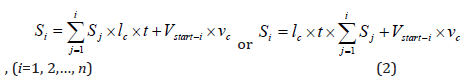where Si is the cost from start node to the present finding node; Sj is the distance between (j-1)th node and jth node, m; lc is the operating cost unit of the main development roads, won/m∙year; t is the lifetime of the main development roads, year; Vstart-i is an amount of construction work from the start node to the present finding node, m3; vc is the cutting and filling cost unit, won/m3.where z is the z coordinate value of area (xj-1, yj-1)-(xj, yj); i is number of the present node on the path list.

The predicting cost Di is calculated in the cost and from the present finding node to the target node. That is,where vi and vend are the distance from start node to the present finding node and the target node, respectively; Vi-end is an amount of construction work from ith node to the target node, m3.

The sum of cost in the present finding node from the above equation is calculated as follows: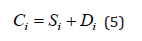Also, in this paper, the constraint conditions which should be considered in searching the route of the main haul roads using A* algorithm are defined as follows;

Firstly, the gradient of the main haul roads should be less than the maximum longitudinal gradient and the code of gradient should be unchanged. It is related to be ensured the safety of haul work. Unchanged code of gradient means that if the gradient of road is started with positive code, it should be unchanged to the last, and if with negative code, it should be remained with “-” code to the last, because if the code of gradient is changed in the path, the haul distance can be longer.

Secondly, after the gradient interval, there should be sure the easement interval because the lifetime of engine may be reduced by overcoming the gradient interval. In other words, in order to ensure the safety of the main development roads, there should be the easement interval whenever it has either positive gradient or negative gradient.

Consequently, by combining the cost function of A* algorithm with the constraint conditions which should be considered in the path finding, we developed the modified A* algorithm to search the route of the main haul roads for developing surface mine with the serious topographic relief.

Based on the constraint conditions mentioned above, the schematic diagram of path finding by new A* algorithm is shown in Figure 1 as an example. Here, the optimal path is defined as the path D2 through the point Pi and Q2 (Figure 1).

The new algorithm in detailed is established as follows:

Step 1. Mine area terrain to be developed is digitized by Geovia Surpac 6.6 software.

The digitized terrain data are the *.str file with Surpac file format. x, y and z coordinate array of all the points on terrain of surface mine area to be developed is made by exporting *.str file data and it is used as the data field.

Step 2. Determine the two endpoints of the main haul roads to be constructed and input the x, y and z coordinates of two endpoints of the main development roads. The two endpoints of the main haul roads are determined around the open-pit mining field, overburden pile, crushing plant or explosive warehouse or on the already built road in the development area.
Step 3. Input the reference data for the design of the main development roads. In this stage, input the average and maximum value of longitudinal gradient of the main haul roads and the interval between terrain contours. And determine the theoretical length of the main haul roads using the average and maximum longitudinal gradient of the main development roads, the z coordinates of start node and target node.
Step 4. Search the path using A* algorithm. First, estimate whether the neighbour node of finding position is in the gradient interval or in the easement interval. If the node neighboured to the present finding point is in the easement interval, the next finding node is selected as node with the same z value until the length of easement interval according to the velocity of haul vehicles is satisfied. If the node neighboured to the present finding point is in the gradient interval, the next node is selected as node with the elevation according to the longitudinal gradient. And then, using above Equations. (2)-(5), calculate Si, Di and Ci of neighbour nodes satisfied judgment conditions of present finding node and select the lowest cost node among them and add it in the path node array, and search continuously.
Step 5. Finish the finding when the coordinate of target node is in the nodes array of path.
Step 6. Save the path.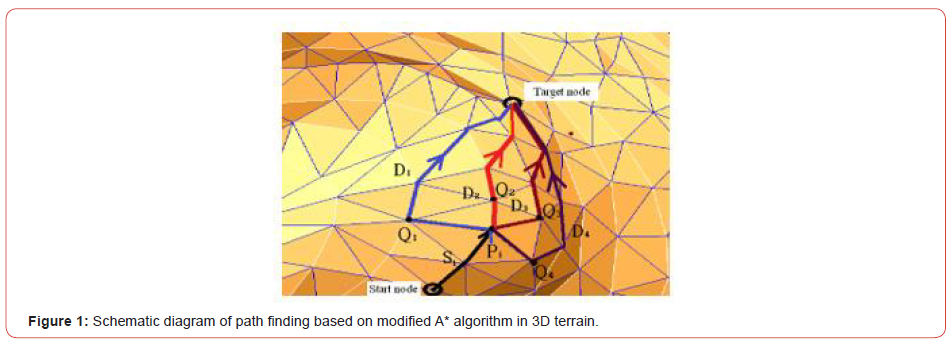##### Comparative estimation between modified A* algorithm and GA

In this study, we have compared A* algorithm with GA on the same opening-up project to analyse the advantages and disadvantages of proposed method. GA is stochastic search techniques inspired in the natural process evolution of species guided by the principle of the survival of the fittest. GA iteratively evolves a population of individuals representing candidate solutions of the optimization problem. The evolution process involves the probabilistic application of operators to find better solutions . The solution of an optimization problem by GA starts with population random strings denoting several design vectors. The population size in GA is mutually fixed. Each string is evaluated to find its fitness values.

The population is operated by 3 operators: reproduction, crossover and mutation to produce a new population of points or design.

Reproduction is first operation applied to the population to select good string from pool. The reproduction operator is also called the selection “operator”. In a commonly used reproduction operator, a string is selected from the pool with probability proportional to its fitness. After reproduction, crossover is implemented to create new strings by exchanging information among strings of mating pool. Child fitness is higher than parents. The crossover is the main operator by which new string with better fitness values are created for new generation. So, we can say that reproduction operator selects good string for mating pool, the crossover operator recombines the substring of good strings of the mating pool to create string and mutation operator alters the string locally. The use of 3 operations successfully finds new generation.

Advantage of using GA is that it does not have much mathematical requirements about the optimization problems due to their evolutionary nature . The evolution operators make GA effective at performing global search. GA for searching path of main haul roads in opening-up area of the surface mine is as follows.

Step 1. Prepare terrain data of ore deposit area.
Step 2. Input two endpoints of road.
Step 3. Consider a road route as an individual and input the number of individual and road route.
Step 4. Form a population of individuals by various routes between two endpoints.
Step 5. Evolve a population of individuals to 100 generations by reproduction, crossover and mutation.

At this time, reproduction probability is calculated according to fitness function fk of the kth individual as follows:where Pk is reproduction probability of the kth individual; M is the number of individuals in a population.
Fitness function fk for selection is as follows: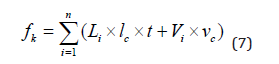where Li is the ith interval length of road route, m; lc is the operating cost unit of the main development roads, won/m∙year; t is the lifetime of the main development roads, year; Vi is an amount of construction work of ith interval, m3; vc is the constructing cost unit, won/ m3; n is the number of nodes in one road route. Fitness for finding optimal route is calculated as follows:where Uk is fitness of the kth individual; fmax and fmin are the maximum and minimum value of fitness function, respectively.

Step 6. Calculate the fitness by Equation (8) and reproduce an individual that the fitness is higher and select an individual that the fitness is lower according to reproduction probability by Equation (6).
Step 7. Perform crossover with two individuals
Step 8. Mutate the string according to mutation probability.
The above operation is repeated until the stop criterion is satisfied as follows:The individual corresponding to the maximum fitness given by the above operation is the optimal route of main development roads.

Figure 2 shows the results of route finding between № 1 openpit and dump by using GA and A* algorithm in conditions lc=2 000 won/m∙year; t=5 year; vc= 320 won/ m3, respectively. While Table 1 shows the coordinate values of searching nodes and route length and execution time by using two methods (Figure 2) (Table 1).

Table 1:Comparative estimation of route-finding results using A* algorithm and GA.As shown in Figure 2 and Table 1 above, it can be found that route finding result using A* algorithm is better than GA, with shorter route length of 94 m.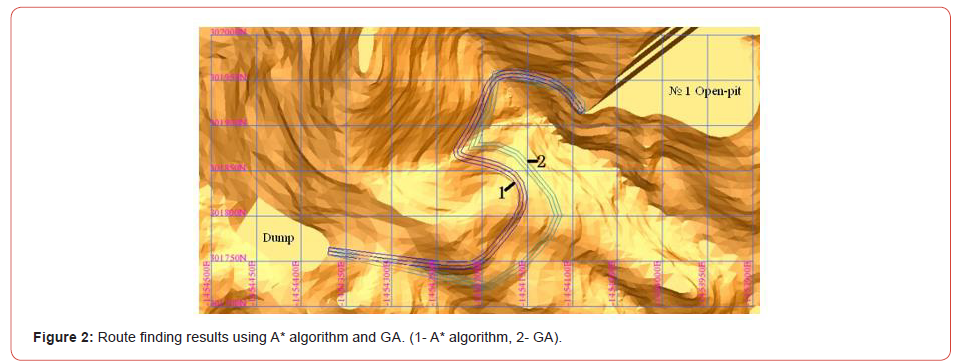#### Application Case

We have introduced the proposed method in development design of a limestone surface mine for Kangdong cement factory in Pyongyang, DPR of Korea. As the limestone deposit area is a mountainous deposit which the relief of terrain is heavy, it is located at 1°53′ E longitude and 39°6′ S latitude. The highest elevation in the study area is +155 m above sea level and the lowest elevation is +4 m above sea level.

Figure 3 shows the result digitized the mine terrain using Surpac. From Figure 3, we established the positions such as openpit, dump, explosive warehouse and crashing plant and so on and set up width, slope angle and gradient of main haul roads according to the characteristics of truck (Figure 3).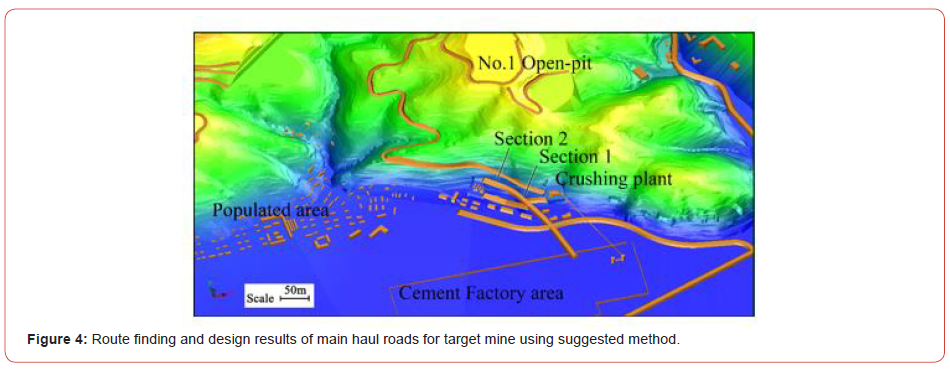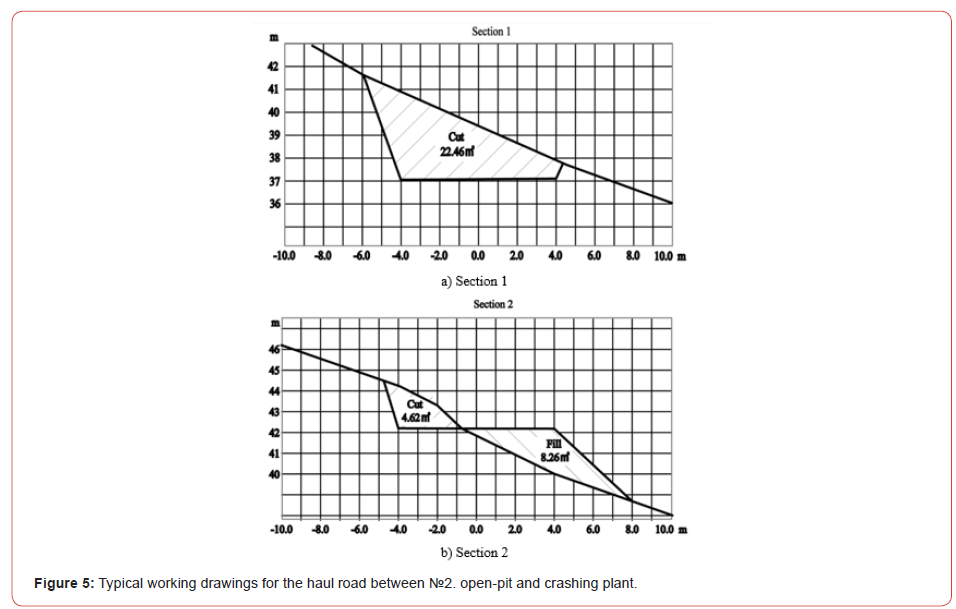#### Conclusion

In this study, we proposed a method to find the optimal route of main haul roads for opening–up the mountainous surface mine using A* algorithm in condition of 3D and to design main haul roads by using Surpac software in 3D environment. The proposed method can resolve the problems that occur when the route of main haul road is determined with the conventional LCPA method only. And this method enables us to find the optimal route of main haul roads for opening–up the mountainous surface mine in the planning and designing stage and in condition of which the main haul roads do not exist at all.

Conclusions obtained from research results above are as follows.
1. Firstly, the optimal route of main haul roads for opening-up the mountainous surface mine can be fully searched by combining the A* algorithm suggested in this paper and Surpac software.
2. Secondly, A* algorithm is more efficient than genetic algorithm (GA) in aspects to the least-cost and execution time for the optimal route finding of main haul roads.
3. Thirdly, this method can be applied to all kinds of the optimal path finding and road design for opening-up the mountainous surface mine.

In the real world, it may still be existed various types of obstruction factors such as hydrogeological condition, protection structures, and rock engineering conditions. Therefore, in future work, it would be interesting to improve the proposed method to consider the river, protection objects, rock condition and so on in finding the optimal route of main haul roads.

#### Data Availability

The data used to support the findings of this study are available from the corresponding author upon request.

#### Acknowledgment

The authors gratefully acknowledge the financial support from the National Science and Technical Development Foundation of DPR Korea (Grant No. 24) and the National Key Basic Research Program of DPR Korea (Grant No. 2019). The authors appreciate the help of many people on this project including the technical staff in Kangdong Cement Factory.

None.

Article Details
Citation
Keywords
Scroll to
Scroll to Top